# Multiway Trees CS 5010 Program Design Paradigms Bootcamp

• Slides: 26Introduction • We've talked about binary trees • Sometimes, we need to construct trees in which each node has an unbounded number of sons. We call these multi-way trees. – example: a file system, in which a directory can have any number of files or directories in it. – example: S-expressions, in which a Lo. SS may contain any number of strings or So. S's. – an XML item. – in this lesson, we'll do a case study of one application of multi-way trees. 2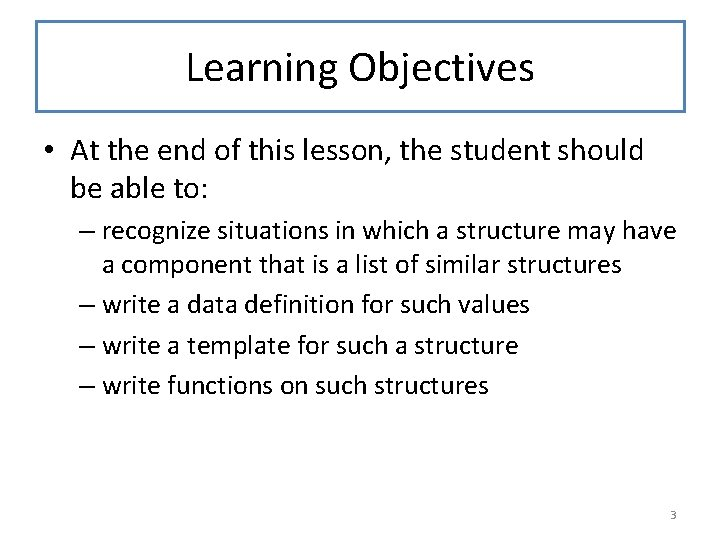Learning Objectives • At the end of this lesson, the student should be able to: – recognize situations in which a structure may have a component that is a list of similar structures – write a data definition for such values – write a template for such a structure – write functions on such structures 3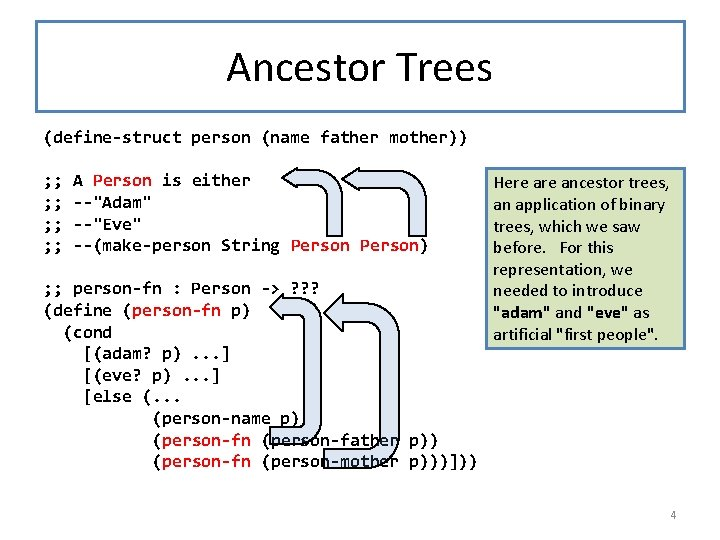Ancestor Trees (define-struct person (name father mother)) ; ; ; ; A Person is either --"Adam" --"Eve" --(make-person String Person) ; ; person-fn : Person -> ? ? ? (define (person-fn p) (cond [(adam? p). . . ] [(eve? p). . . ] [else (. . . (person-name p) (person-fn (person-father p)) (person-fn (person-mother p)))])) Here ancestor trees, an application of binary trees, which we saw before. For this representation, we needed to introduce "adam" and "eve" as artificial "first people". 4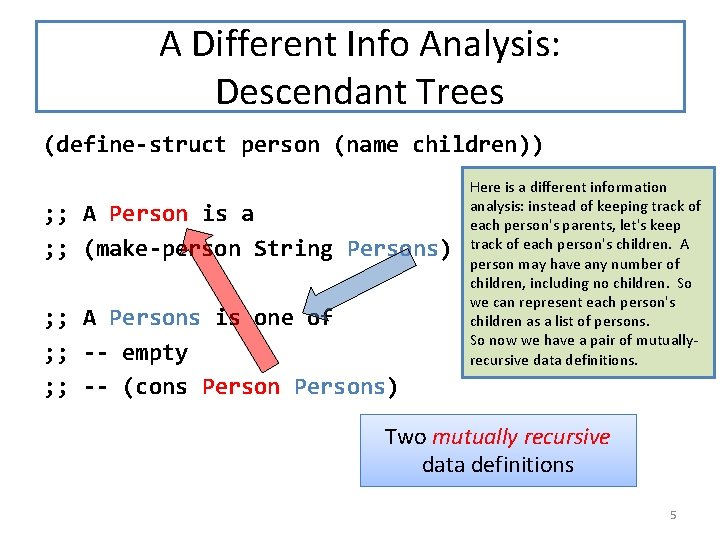A Different Info Analysis: Descendant Trees (define-struct person (name children)) ; ; A Person is a ; ; (make-person String Persons) ; ; A Persons is one of ; ; -- empty ; ; -- (cons Persons) Here is a different information analysis: instead of keeping track of each person's parents, let's keep track of each person's children. A person may have any number of children, including no children. So we can represent each person's children as a list of persons. So now we have a pair of mutuallyrecursive data definitions. Two mutually recursive data definitions 5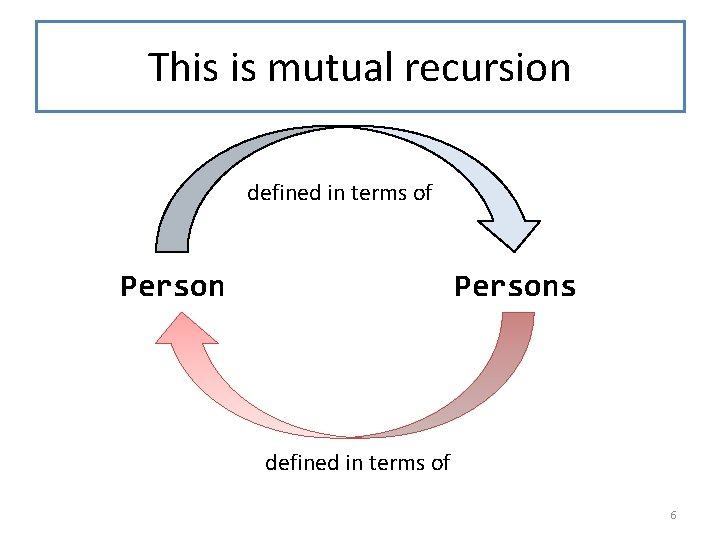This is mutual recursion defined in terms of Persons defined in terms of 6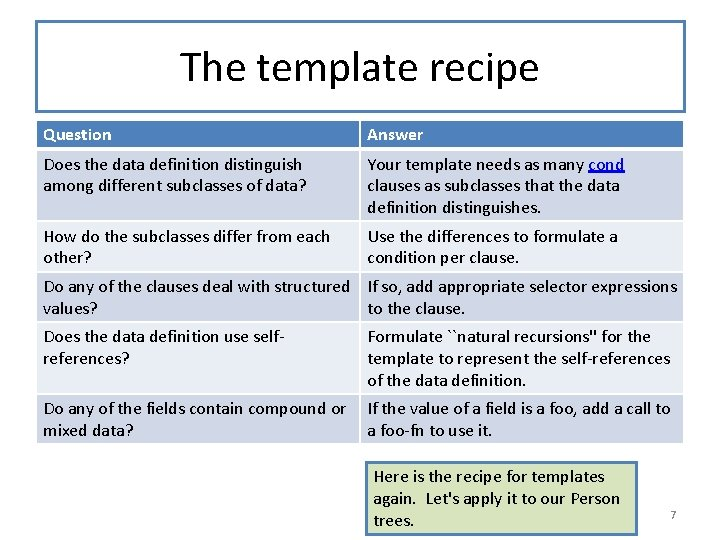The template recipe Question Answer Does the data definition distinguish among different subclasses of data? Your template needs as many cond clauses as subclasses that the data definition distinguishes. How do the subclasses differ from each other? Use the differences to formulate a condition per clause. Do any of the clauses deal with structured If so, add appropriate selector expressions values? to the clause. Does the data definition use selfreferences? Formulate ``natural recursions'' for the template to represent the self-references of the data definition. Do any of the fields contain compound or mixed data? If the value of a field is a foo, add a call to a foo-fn to use it. Here is the recipe for templates again. Let's apply it to our Person trees. 7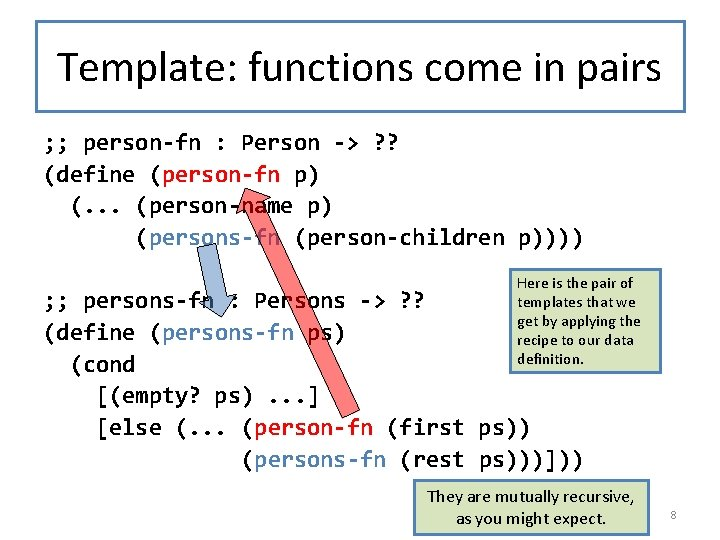Template: functions come in pairs ; ; person-fn : Person -> ? ? (define (person-fn p) (. . . (person-name p) (persons-fn (person-children p)))) Here is the pair of templates that we get by applying the recipe to our data definition. ; ; persons-fn : Persons -> ? ? (define (persons-fn ps) (cond [(empty? ps). . . ] [else (. . . (person-fn (first ps)) (persons-fn (rest ps)))])) They are mutually recursive, as you might expect. 8The template questions And here the Given the answer forare a person’s template as children, how doquestions, we find the usual. answer for the person? ; ; person-fn : Person -> ? ? (define (person-fn p) (. . . (person-name p) (persons-fn (person-children p)))) What’s the answer for ; ; persons-fn : Persons -> ? ? the empty Persons? (define (persons-fn ps) (cond [(empty? ps). . . ] [else (. . . (person-fn (first ps)) (persons-fn (rest ps)))])) Given the answer for the first person in the list and the answer for the rest of the people in the list, how do we find the answer for the whole list? 9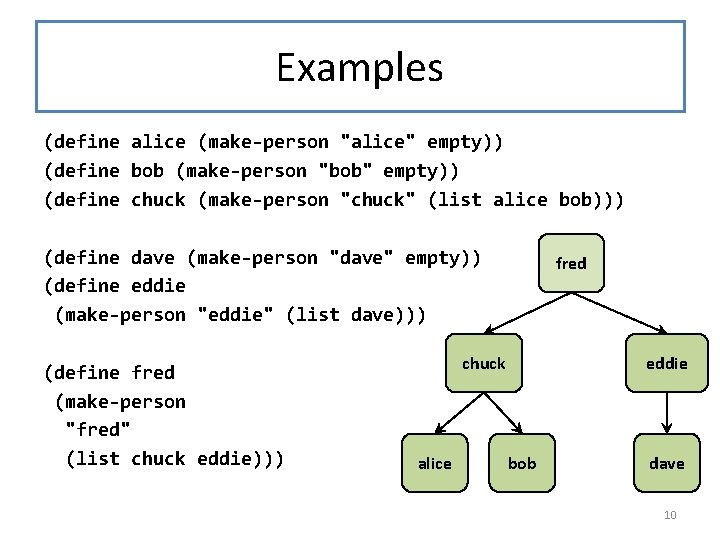Examples (define alice (make-person "alice" empty)) (define bob (make-person "bob" empty)) (define chuck (make-person "chuck" (list alice bob))) (define dave (make-person "dave" empty)) (define eddie (make-person "eddie" (list dave))) (define fred (make-person "fred" (list chuck eddie))) fred chuck alice eddie bob dave 10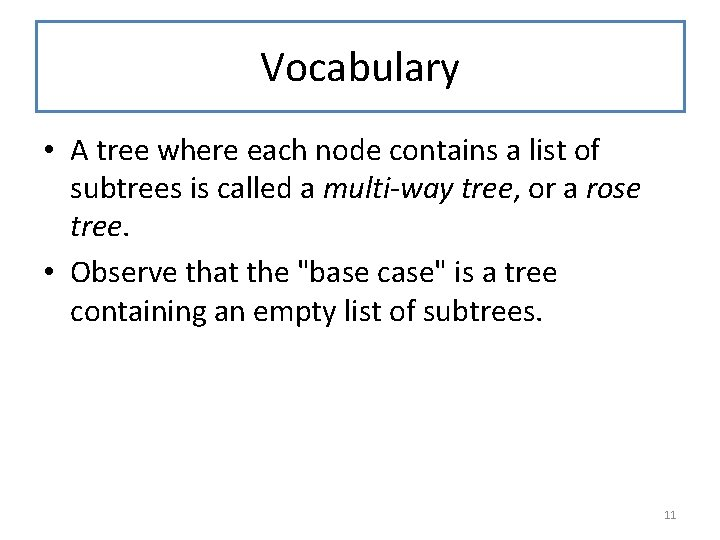Vocabulary • A tree where each node contains a list of subtrees is called a multi-way tree, or a rose tree. • Observe that the "base case" is a tree containing an empty list of subtrees. 11Grandchildren Here's a simple function we might want to write. ; ; grandchildren : Person -> Persons ; ; GIVEN: a Person ; ; RETURNS: a list of the grandchildren of the given ; ; person. ; ; EXAMPLE: (grandchildren fred) = (list alice bob dave) ; ; STRATEGY: Use template for Person on p (define (grandchildren p) (. . . (person-children p))) Q: Given p’s children, how do we find p’s grandchildren? A: We need a function which, given a list of persons, produces a list of all their children 12persons-all-children ; ; persons-all-children : Persons -> Persons ; ; GIVEN: a list of persons ; ; RETURNS: a list of all their children. ; ; (persons-all-children (list fred eddie)) ; ; = (list chuck eddie dave) (define (persons-all-children ps) (cond [(empty? ps). . . empty] ] [else (. . . (append (person-children (first ps)) (persons-all-children (rest ps)))])) This one was too easy! It didn't require mutual recursion. 13Putting it together ; ; grandchildren : Person -> Persons ; ; STRATEGY: Use template for Person on p (define (grandchildren p) (persons-all-children (person-children p))) ; ; persons-all-children : Persons -> Persons ; ; STRATEGY: Use template for Persons on ps (define (persons-all-children ps) (cond [(empty? ps) empty] [else (append (person-children (first ps)) (persons-all-children (rest ps)))])) 14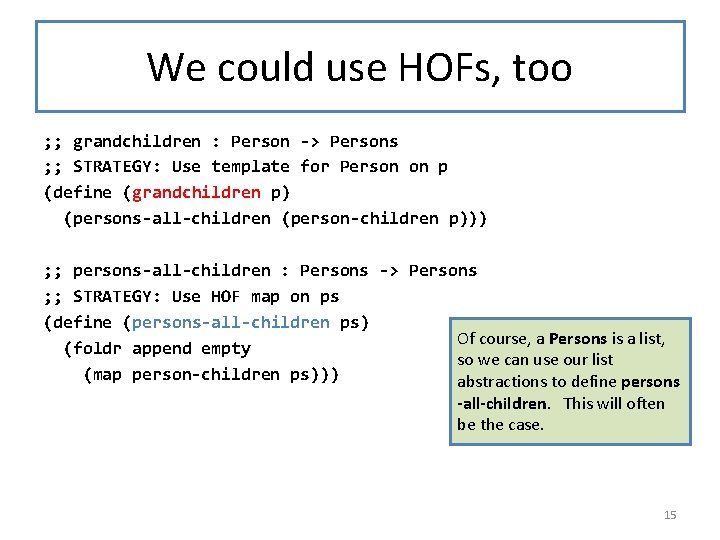We could use HOFs, too ; ; grandchildren : Person -> Persons ; ; STRATEGY: Use template for Person on p (define (grandchildren p) (persons-all-children (person-children p))) ; ; persons-all-children : Persons -> Persons ; ; STRATEGY: Use HOF map on ps (define (persons-all-children ps) Of course, a Persons is a list, (foldr append empty so we can use our list (map person-children ps))) abstractions to define persons -all-children. This will often be the case. 15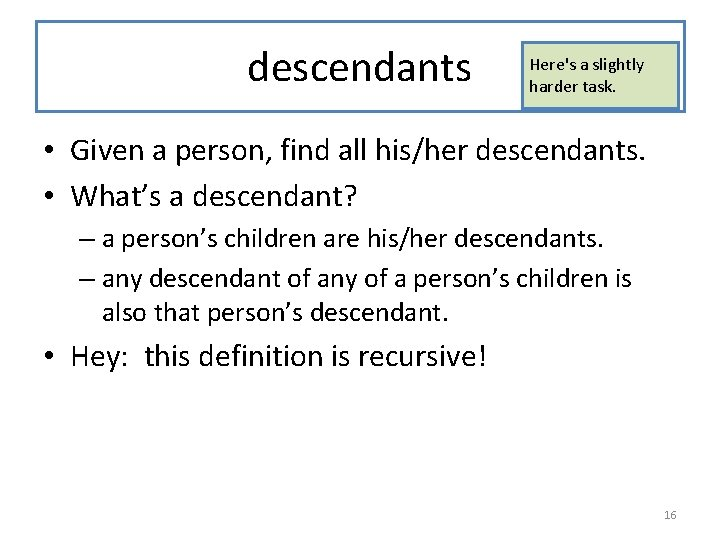descendants Here's a slightly harder task. • Given a person, find all his/her descendants. • What’s a descendant? – a person’s children are his/her descendants. – any descendant of any of a person’s children is also that person’s descendant. • Hey: this definition is recursive! 16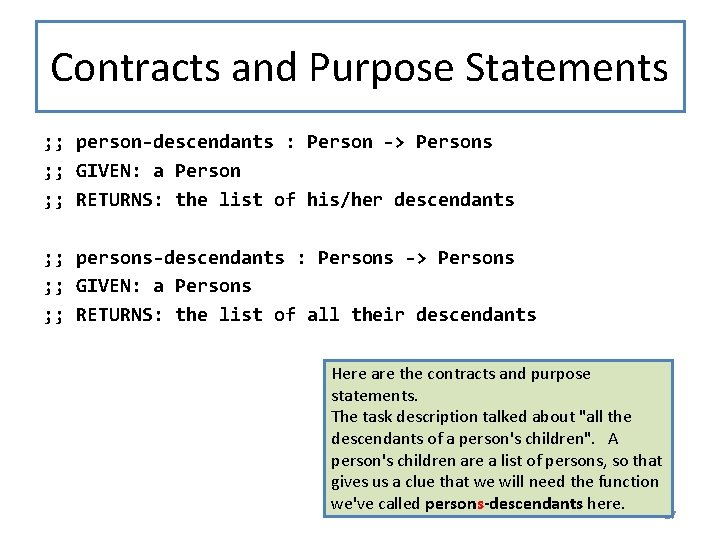Contracts and Purpose Statements ; ; person-descendants : Person -> Persons ; ; GIVEN: a Person ; ; RETURNS: the list of his/her descendants ; ; persons-descendants : Persons -> Persons ; ; GIVEN: a Persons ; ; RETURNS: the list of all their descendants Here are the contracts and purpose statements. The task description talked about "all the descendants of a person's children". A person's children are a list of persons, so that gives us a clue that we will need the function we've called persons-descendants here. 17Examples fred chuck alice eddie bob dave (person-descendants fred) = (list chuck eddie alice bob dave) (persons-descendants (list chuck eddie)) = (list alice bob dave) 18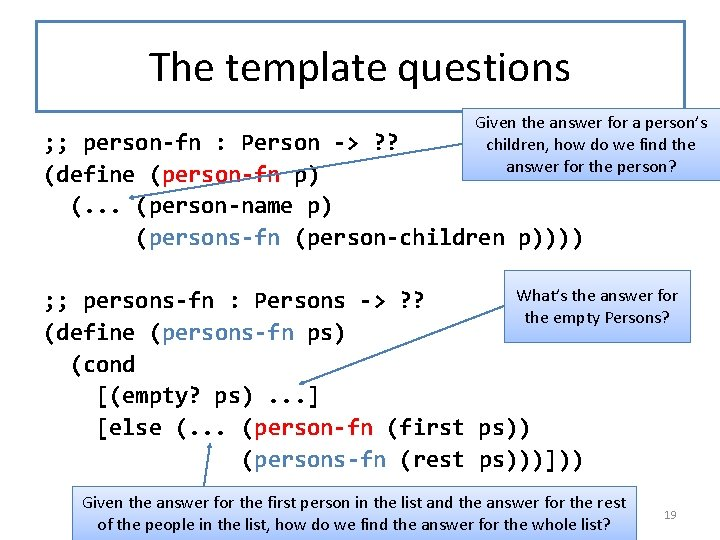The template questions And here the Given the answer forare a person’s template as children, how doquestions, we find the usual. answer for the person? ; ; person-fn : Person -> ? ? (define (person-fn p) (. . . (person-name p) (persons-fn (person-children p)))) What’s the answer for ; ; persons-fn : Persons -> ? ? the empty Persons? (define (persons-fn ps) (cond [(empty? ps). . . ] [else (. . . (person-fn (first ps)) (persons-fn (rest ps)))])) Given the answer for the first person in the list and the answer for the rest of the people in the list, how do we find the answer for the whole list? 19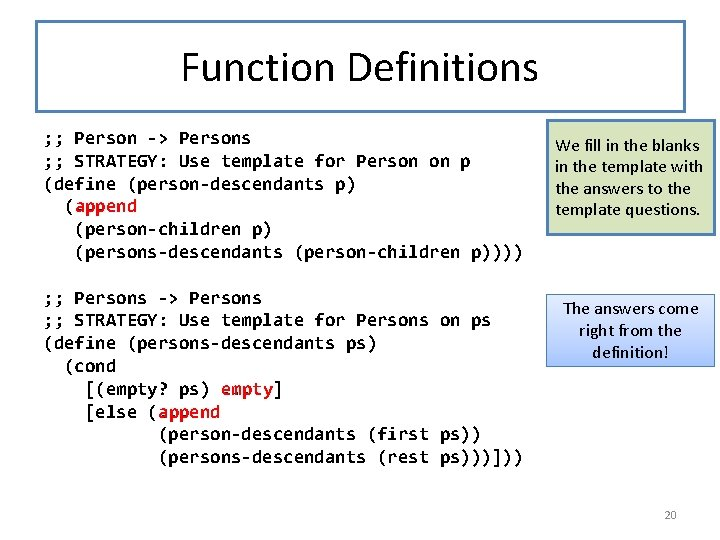Function Definitions ; ; Person -> Persons ; ; STRATEGY: Use template for Person on p (define (person-descendants p) (. . . (append (person-children p) (persons-descendants (person-children p)))) ; ; Persons -> Persons ; ; STRATEGY: Use template for Persons on ps (define (persons-descendants ps) (cond [(empty? ps). . . empty] ] [else (append (. . . (person-descendants (first ps)) (persons-descendants (rest ps)))])) We fill in the blanks in the template with the answers to the template questions. The answers come right from the definition! 20Or, with the HOFs ; ; Person -> Persons ; ; STRATEGY: Use template for Person on p (define (person-descendants p) (append (person-children p) (persons-descendants (person-children p)))) ; ; Persons -> Persons ; ; STRATEGY: Use HOF map followed by foldr (define (persons-descendants ps) As we did before, we could replace the structural (foldr append empty (map person-descendants ps))) decomposition on Persons with Higher-Order Function Composition. The functions are still mutually recursive. 21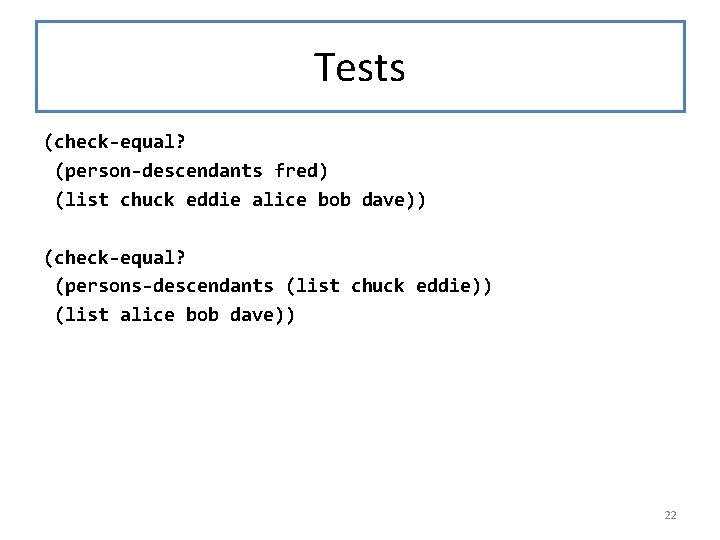Tests (check-equal? (person-descendants fred) (list chuck eddie alice bob dave)) (check-equal? (persons-descendants (list chuck eddie)) (list alice bob dave)) 22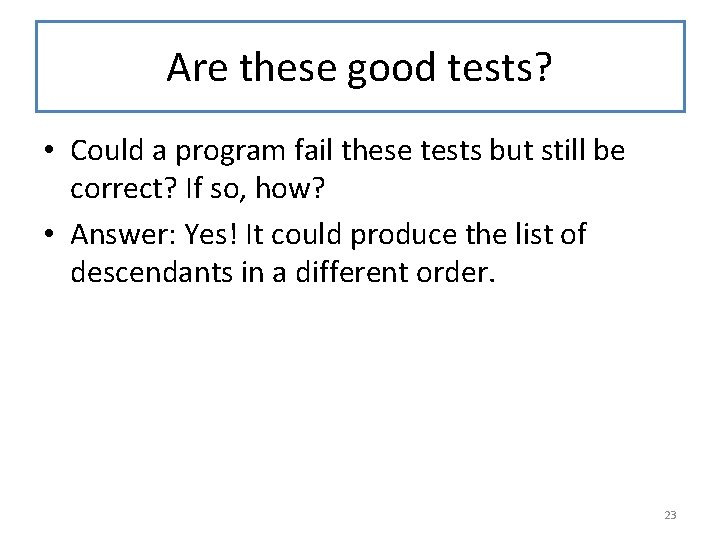Are these good tests? • Could a program fail these tests but still be correct? If so, how? • Answer: Yes! It could produce the list of descendants in a different order. 23Better Tests (require "sets. rkt") ; ; or whatever. . . (check set-equal? (person-descendants fred) (list chuck eddie alice bob dave)) (check set-equal? (person-descendants fred) (list chuck eddie alice dave bob)) (check set-equal? (persons-descendants (list chuck eddie)) (list alice bob dave)) There are two ways we could solve this problem: 1. We could have our purpose statement specify the order in which the descendants are to be listed. 2. We could use smarter tests that would accept the answer list in any order. Here we've adopted the second approach. Instead of checkequal? , we use check, which takes as its first argument a predicate to be used to compare the actual and expected answers. We'll have to require a library that provides set-equal? -- the file sets. rkt, which we worked with last week, will do nicely. We've put a working copy of sets. rkt in the Examples file for this week. Here are some tests for (descendants fred) that list the answer in two different orders. 24Summary • You should now be able to: – recognize situations in which a structure may have a component that is a list of similar structures – write a data definition for such values – write a template for such a structure – write functions on such structures 25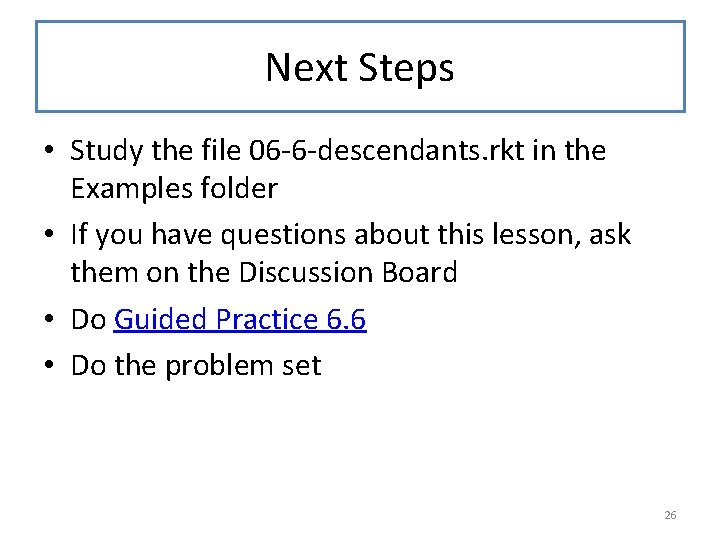Next Steps • Study the file 06 -6 -descendants. rkt in the Examples folder • If you have questions about this lesson, ask them on the Discussion Board • Do Guided Practice 6. 6 • Do the problem set 26